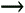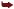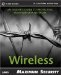# Key Extraction

So far we have illustrated serious theoretical weaknesses in WEP. However, in practice, the preceding examples are difficult to implement. Although it is possible to extract clear text from the encrypted information through the use of a series of XOR calculations, the amount of information obtained can be limited. A hacker would have to completely saturate a WLAN with known data until every IV combination is known. Then the hacker would have to create a program that could decipher each encrypted packet by XORing it with its associated plaintext value. This is not easily accomplished because of the inclusion of extraneous data with WLAN packets. However, what if a hacker could use the preceding weaknesses in such a way that she could extract the password from the WLAN traffic? If a hacker knew the password, she could connect to a WLAN and become a " legitimate " user of the wireless network.

Unfortunately, this scenario happens all too often in real life. Because of IV collisions and the fact that WLAN packets include several known values such as IP headers, IPX headers, SNAP headers, and more, hackers can deduce parts of the password from the encrypted WLAN data. This weakness has resulted in several WEP cracking programs written to demonstrate this weakness. (In fact, Anton T. Rager, who released the first public code to crack WEP, is himself a technical reviewer of this book.) These programs are discussed in a later chapter. However, for now let's take a closer look at the underlying process.

#### Technical Explanation

There are several tools available online that can be used to crack WEP. Regardless of the tool, they all use the same basic concepts to derive the WEP key. However, some tools integrate more complex algorithms and guessing methods in addition to the standard cracking technique. Although these achieve the goal in a shorter period of time because of a reduced number of required packets, they also rely on a larger probability factor, which can lead to false positives.

In short, almost every packet sent over the WLAN includes a value known as a SNAP header . This value (0xaa) is almost always the first plaintext byte that is XORed with the first PRGA byte to produce the first ciphertext byte. As we discovered in the previous example, if you know two of the values passed into or out of a XOR operation, you can derive the third. Using this method, in addition to a weakness in the initialization of the KSA, crackers have discovered a way to process captured information and extract a probable password, byte by byte. Given enough packets (2,000,000 “5,000,000), the probability is reduced drastically, and the actual key is revealed within a very small margin of error.

This method relies on the fact that a packet containing an IV with a certain format has a relatively high (5%) chance of revealing a byte of the password, as explained in the next section. In addition to this, every packet sent over the WLAN contains a known value (the SNAP header) that is used to define the connection type. This header always has the value 0xAA in hex, which can be used in an XOR calculation, as previously demonstrated, to deduce the password.

It only takes roughly 2,000 packets containing a weak IV before a hacker can produce the password. However, it should be noted that several million packets will have to be sent through the WLAN and subsequently filtered by a hacker to find this number of weak IVs.

#### The IV

As previously discussed, the format of the weak IV is as follows : (B+3, N-1, X)

` IV  1  IV  2  IV  3  B+3          N-1           X `

In this format:

B = Byte of password being guessed. (Recall from previous discussions that the password is actually the IV combined with the shared password.) Because we know the first three characters of the password, we do not need to guess them.

N = Index value, which is typically 256 in most WEP devices.

X = Any value from 0 “255. This is because any ASCII, decimal, or hex value can be written in decimal format, which only contains 256 characters.

Now we will walk through an example of the first four loops of the KSA using a selected IV. We are doing this to determine what the values would be in case we want to test an IV for a weakness. We will also be using these values later in the chapter, as we attempt to reverse-engineer the KSA process ”just as a hacker would when attempting to crack WEP.

#### The WEP/RC4 Algorithms

To encrypt data, WEP uses the RC4 algorithm. This algorithm is one of the most well-known and used forms of encryption currently available. The following in-depth and detailed explanation will show how RC4 is used, and abused, by the WEP cracking process. Because WEP relies heavily on RC4, it is important to understand this encryption scheme. Therefore, it is best to start with an explanation of the RC4 algorithm, shown in Table 5.4.

##### Table 5.4. RC4 KSA and PRGA Functions

KSA Function

PRGA Function

KSA

KSA(K)

Initialization: For i = 0 ... N - 1 S[i] = i j = 0 Scrambling: For i = 0 ... N - 1 j = j + S[i] + K[i mod l] Swap(S[i], S[j])

PRGA(K)

Initialization: i = 0 j = 0 Generation Loop: i = i + 1 j = j + S[i] Swap(S[i], S[j]) Output z = S[S[i] + S[j]]

When investigating the weaknesses of WEP, there are several assumptions that need to be made to facilitate the testing process. For our example, we will assume that the following information is true.

The assumed and known values:

• N = 256

• B = 0

• IV = B+3, 255, 7 = 3, 255, 7

• SK = 2, 2, 2, 2, 2 (a preshared password)

• K = IV + SK = 3, 255, 7, 2, 2, 2, 2, 2

• l = the number of elements in K = 8

• Assume that no S elements are swapped when i > B + 3 (5% probability of happening)

• S [i] = State array with 256 consecutive values (0 “255) as required in initialization of KSA (see Table 5.4).

#### IV + Preshared Password Array Values

The following depicts how the secret key (IV + preshared password) value is converted into an array for use by the algorithm. The array is labeled K for Key, and holds eight values; three from the IV, and five from the shared password.

` K    =3   K    =255   K    =7   K    =2   K    =2   K    =2   K    =2   K    =2 `

#### KSA 1 (Loop 1)

The first step in the RC4 algorithm is to set up the state array. Because we assumed this array, which holds 256 values, has already been seeded , the next step is to start to randomize the order in which the array has stored these values. In other words, we must scramble the values held by the array to ensure it is impossible to know what value is held in any position of the array.

To start, we list the known and assumed values held by the S array (State array), and by the i and j variables .

` i=0   j=0   S    =0   S    =1   S    =2   S    =3  S    =255 `

Next, we recalculate the value of j using the following equation, which uses the values of the i , j , and S array values listed in the preceding.

` j=j + S  [i]  + K  [i mod l]  = 0 + S    + K    = 0 + 0 + 3 = 3j = 3 `

Now that j holds a new value (3), we then perform a Swap calculation using the following format. In this calculation, the values of i and j are used to determine what positions of the S array are swapped. In this example, i=0 and j=3 , which means the values held by S  and S  are swapped with each other.

` Swap (S  [i]  , S  [j]  )Swap (S    , S    )S    = 0, S    = 3S    = 3, S    = 0 `

#### KSA 2 (Loop 2)

Now that we've worked through the KSA algorithm once, we need to do it a few more times to properly set up the KSA to the point where we can demonstrate its weakness. See if you can work along with the following algorithm.

` i=1         j=3            S    =3      S    =1        S    =2      S    =0  S    =255  j=j + S  [i]  + K  [i mod l]  = 3 + S + K[1 mod 8] = 3 + 1 + 255 = 259 mod 256 = 3j = 3  Swap(S[i], S[j])Swap (S, S)S=1, S=0S=0, S=1 `

#### KSA 3 (Loop 3)

` i=2         j=3             S  =3    S=0      S=2     S=1  S    =255  j=j + S  [i]  + K  [i mod l]  = 3 + S + K = 3 + 2 + 7 = 12j = 12  Swap(S[i], S[j])Swap (S, S)S=2, S=12S=12, S=2 `

NOTE

So far we have still not used any elements of the original password (22222)! In other words, anyone has the ability to reproduce the values generated by the first three iterations through the KSA loop. This, in combination with the 5% chance that the S [i] values for the S [0 ”> 3] (S, S, S, S) will not change, is the very heart of WEP's weakness. In addition, the fourth KSA iteration always assigns S  with a value related to the byte of the password being attacked .

#### KSA 4 (Loop 4)

At this point, we are going to continue working through the algorithm as the program would. A hacker would not be able to do this, simply because he does not yet know the value of the password. However, this information will help you understand the reverse process that the hacker must go through to reverse-engineer the password from the WEP algorithm.

` i=3        j=12           S=3        S=0        S=12   S=1  S    =255  j=j + S  [i]  + K  [i mod l]  = 12 + S + K = 12 + 1 + 2 = 15  Swap(S[i], S[j])Swap (S, S)S=2, S=8S=15, S=1 `

#### KSA 4

` Values at i=3 Outputi=3  j=8  S=3 S=0  S=12 S=15  S=255 `

At this point, we can stop processing the KSA loop. (Although there are other situations in which the KSA could be predicted , their probability is minimal, and is beyond the scope of this chapter. We are simply illustrating the major weakness in WEP.)

The next step is to calculate the first PRGA output byte based on the computed KSA.

#### PRGA 1

The PRGA is the second of two algorithms that WEP/RC4 uses to encrypt data. It starts by initializing two variables: i and j . It then uses these variables in conjunction with the values held in the now scrambled S array to produce a streaming key that is used in the final XOR calculation that actually encrypts the data. This algorithm also uses the swapping technique previously used by the KSA.

` i=3     j=8     S=3     S=0      S=12      S=15  S=3 `

The preceding are the values held in the S array positions, assuming that they were not altered during the KSA algorithm (5% chance). These values will be used by the PRGA.

` i=0         j=0  i = i + 1 = 0 + 1 = 1  j = j + S[i] = 0 + S = 0 + 0 = 0  Swap(S[i], S[j])Swap (S, S)S=0, S=3S=3, S=0  z = S[S[i] + S[j]] = S[S + S] = S[3 + 0] = S = 15 `

We now have the first PRGA byte (15). This byte, if you recall from previous discussions, is XORed with the first plaintext byte to produce the first ciphertext byte. As you have also learned, the first byte of plaintext is almost always the SNAP header, which is equal to 0xAA (hex).

The following illustrates this calculation. Note that we are XORing a decimal value and hex value. You can do the same with any scientific calculator, including the one provided free with your operating system.

` Ciphertext Byte  1  = z  XOR  Plaintext Byte  1  = 15 (Dec)  XOR  0xAA (Hex) = 165 (Dec) `

Now that we have analyzed what the first output byte would be if we used the password of 22222, let's look at this from a hacker's point of view. A hacker would not know the value of the shared password, which is why he would be cracking WEP. However, the hacker does know the value of the first output byte, the value of the first byte of plaintext (0xAA), and the first three values of the "IV + password" value. By combining this information, the hacker can easily deduce the first output value of the PRGA (z), and thus the value of the password byte used to seed the KSA, with 5% accuracy. To illustrate this, let's examine the steps a hacker would have to go through to extract a byte of the password from a captured IV.

Known Values:

` IV = 3, 255, 7  Ciphertext Byte  1  = 165 (Dec) `
1. Calculate first PRGA output byte (z).

z = 0xAA XOR Ciphertext byte1 = 0xAA (Hex) XOR 165 (Dec) = 15z = 15

From this, we can assume that at the output of KSA 4 , S  equaled 15. The next step is to reproduce the first three iterations of the KSA using the first three values of the IV + preshared password for KSA (1 “3) .

2. Calculate KSA for password 3, 255, 7, ?, ?, ?, ?, ? at times i=0, i=1, i=2.

(See previous example for steps i=0, i=1, i=2)

At the end of KSA 2 , we know the output of KSA 2 based on only the IV and an understanding of the KSA.

#### KSA 2 Output

` i=2     j=3     S=3     S=0     S=2      S=1 `

In addition, we also know the output of KSA 4 as calculated from the deduced PRGA 1 value and an understanding of the KSA.

1. Reverse-engineer the KSA 4 output based on known z value and known KSA 2 output values.

#### KSA 4 Output

[View full width]
`  [View full width]  i=3             j=15             S=3            S=0               S=12S=1  S    =15, S    =S  t-1S    =15, S    =1Swap (S    , S    )S    =1, S    =15  j=j + S  [i]  + K  [i mod 256]  = 15 + S    + K    = 12 + 1 + K    = 15K    = 15  12  1 = 2 `

Therefore: K  = 2

Note that this will not work every time with every value. In fact, there is only a 5% chance this will work. The previous example used a value that we knew would produce the desired results. If you attempt to do this on another value, you have a 1 in 20 chance of obtaining a valid password value. For example, the IV 3, 255, 10 will not produce a valid password byte value.

To determine what values are valid, you must collect and test many possible IVs. This is simply a matter of rote-testing each and every correctly formatted IV (B+3, 255, X) against all the other possible combinations of the formatted IV (B+3, 255, 0 “255). This will result in password leakage, and the capability to rank the IVs based on calculated password byte values. After all the possible IVs are tested , the password byte that is produced the most often wins!Maximum Wireless Security
ISBN: 0672324881
EAN: 2147483647
Year: 2002
Pages: 171

Similar book on Amazon